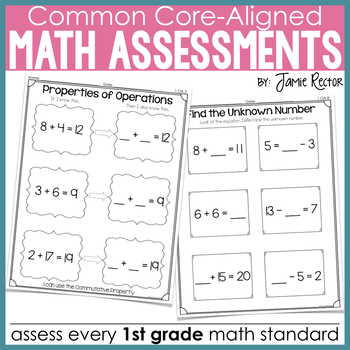# Common Core Standards Math Quick Assessments 1st Grade1st
Subjects
Standards
Resource Type
Formats Included
• PDF
Pages
42 pages

### Description

I have dissected the 1st Grade Common Core Math Standards and created a resource that is perfect for assessing your students! The quick assessments included in this packet will provide formative assessments that are an easy form of documentation for mastery of the Common Core Standards for 1st Grade.

Each quick assessment highlights the specific vocabulary from the standard and some standards even have up to 4 different assessments to target each specific part of the standard. NOTHING is left out of this resource pack! Using this pack of quick assessments will ensure that you have taught and the students have mastered the Common Core Standards in Math in all 4 Domains: Operations and Algebraic Thinking, Numbers and Operations in Base Ten, Measurement and Data, and Geometry.

This product is aligned to the First Grade Common Core Standards for Math.

You might also be interested in:

Interested in a COMPLETE SYSTEM for assessing students in reading using ANY text?

Jamie Rector from 2nd Grade Stuff

Credits:

The Common Core Standards were developed and written by the NGA.

Total Pages
42 pages
N/A
Teaching Duration
1 Year
Report this Resource to TpT
Reported resources will be reviewed by our team. Report this resource to let us know if this resource violates TpT’s content guidelines.

### Standards

to see state-specific standards (only available in the US).
Use addition and subtraction within 20 to solve word problems involving situations of adding to, taking from, putting together, taking apart, and comparing, with unknowns in all positions, e.g., by using objects, drawings, and equations with a symbol for the unknown number to represent the problem.
Solve word problems that call for addition of three whole numbers whose sum is less than or equal to 20, e.g., by using objects, drawings, and equations with a symbol for the unknown number to represent the problem.
Apply properties of operations as strategies to add and subtract. If 8 + 3 = 11 is known, then 3 + 8 = 11 is also known. (Commutative property of addition.) To add 2 + 6 + 4, the second two numbers can be added to make a ten, so 2 + 6 + 4 = 2 + 10 = 12. (Associative property of addition.)
Understand subtraction as an unknown-addend problem. For example, subtract 10 – 8 by finding the number that makes 10 when added to 8.
Relate counting to addition and subtraction (e.g., by counting on 2 to add 2).